The equations of time-harmonic linear elasticity

The aim of this tutorial is to demonstrate the solution of the time-harmonic equations of linear elasticity in cartesian coordinates. These equations are useful to describe forced, time-harmonic oscillations of elastic bodies.

 Acknowledgement: The implementation of the equations and the documentation were developed jointly with David Nigro.

# Theory

Consider a linearly elastic body (of density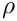, Young's modulus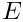and Poisson's ratio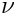), occupying the region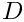whose boundary is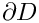. Assuming that the body performs time-harmonic oscillations of frequency of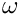its motion is governed by the equations of time-harmonic linear elasticity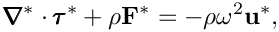where the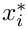are the cartesian coordinates, and the time-periodic stresses, body force and displacements are given by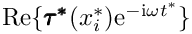,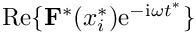and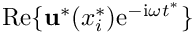respectively. Note that, as usual, the superscript asterisk notation is used to distinguish dimensional quantities from their non-dimensional counterparts where required.

The body is subject to imposed time-harmonic displacements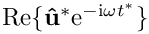along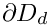, and to an imposed time-harmonic traction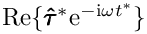along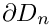where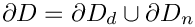. This requires that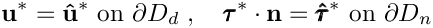where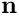is the outer unit normal on the boundary.

The stresses and displacements are related by the constitutive equations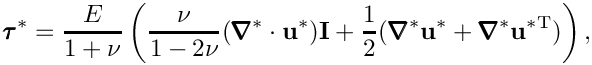where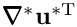represents the transpose of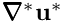.

We non-dimensionalise the equations, using a problem specific reference length,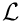, and a timescale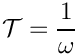, and use Young's modulus to non-dimensionalise the body force and the stress tensor: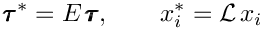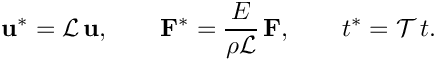The non-dimensional form of the equations is then given by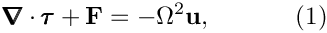with the non-dimensional constitutive relation,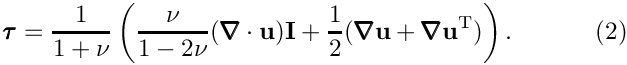The non-dimensional parameter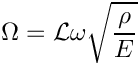is the ratio of the elastic body's intrinsic timescale,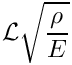, to the problem-specific timescale,, that we used to non-dimensionalise time.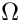can be interpreted as a non-dimensional version of the excitation frequency; alternatively/equivalently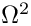may be interpreted as a non-dimensional density. The boundary conditions are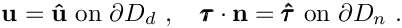# Implementation

Within oomph-lib, the non-dimensional version of equations (1) with the constitutive equations (2) are implemented in the TimeHarmonicLinearElasticityEquations<DIM> equations class, where the template parameter DIM indicates the spatial dimension. Following our usual approach, discussed in the (Not-So-)Quick Guide, this equation class is then combined with a geometric finite element to form a fully-functional finite element. For instance, the combination of the TimeHarmonicLinearElasticityEquations<2> class with the geometric finite element QElement<2,3> yields a nine-node quadrilateral element. As usual, the mapping between local and global (Eulerian) coordinates within an element is given by,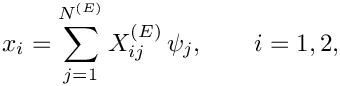where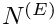is the number of nodes in the element,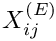is the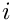-th global (Eulerian) coordinate of the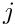-th Node in the element, and the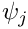are the element's shape functions, defined in the geometric finite element.

All the constitutive parameters are real. The two components of the displacement field have a real and imaginary part. We store the four real-valued nodal unknowns in the order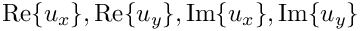and use the shape functions to interpolate the displacements as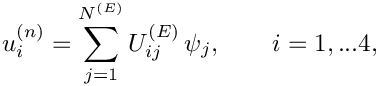where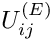is the-th displacement component (enumerated as described above) at the-th Node in the element.

# A test problem: Oscillations of an elastic annulus

We consider the time-harmonic axisymmetric deformation of a 2D annular elastic body that occupies the region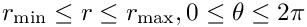, shown in the left half of the sketch below. We impose a constant-amplitude axisymmetric displacement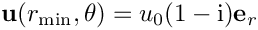on the inner boundary and a constant-amplitude pressure load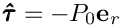on the outer boundary. (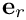is the unit vector in the radial direction).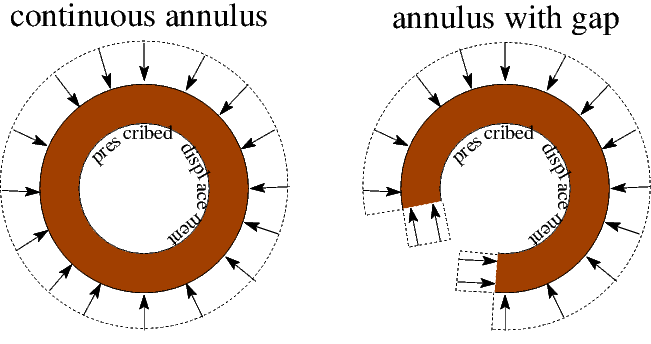Sketch of the test problems.

It is easy to find an analytical solution of this problem by working in polar coordinates and exploiting the axisymmetry of the solution by writing the displacement as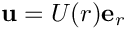. The radial displacement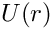is then governed by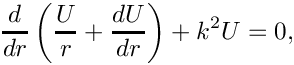where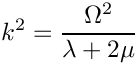and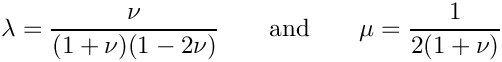are the non-dimensional Lame parameters. The solution of this equation is given by: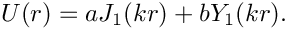where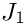and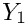are Bessel functions of the first and second kind, respectively. The amplitudes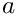and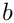can be found using the boundary conditions on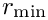and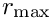. In the driver code discussed below, the (lengthy) expressions forandin terms of the problem parameters can be found in the  GlobalParameters::exact_u()  function.

We note that even though a relatively simple analytical solution (in polar coordinates!) exists for this problem, it is a non-trivial test case for our code which solves the governing equations in cartesian coordinates. However, to show that we can also compute non-trivial solutions, we also consider the case where the annular region has a "gap" and therefore occupies only a fraction (90%) of the circumference. This creates two additional boundaries (the radial lines bounding the "gap" and we subject these to the same pressure that acts on the outer boundary, as shown in the right half of the sketch above.

# Results

The figures below show "carpet plots" of the real and imaginary parts of the exact (green) and computed (red) horizontal displacement (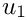– the plots for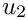obviously look very similar) for the continuous coating and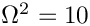,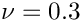,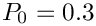,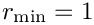and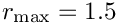.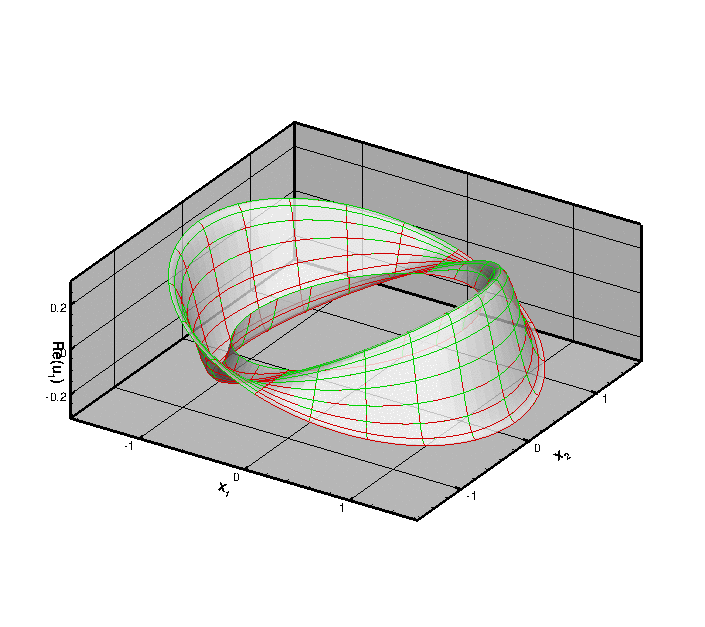Real part of the horizontal displacement. Green: exact; red: computed.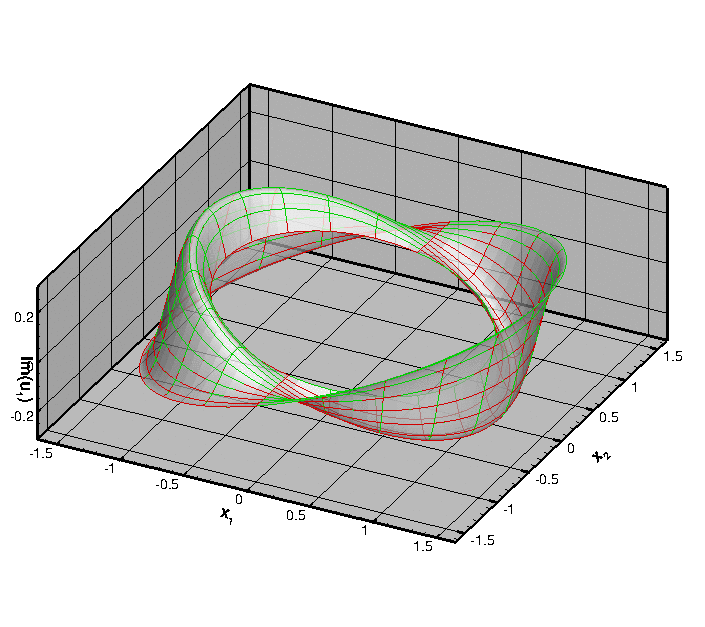Imaginary part of the horizontal displacement. Green: exact; red: computed.

To demonstrate that the resulting displacement field is indeed axisymmetric, here is a plot of the real part of the radial displacement,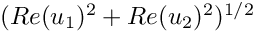.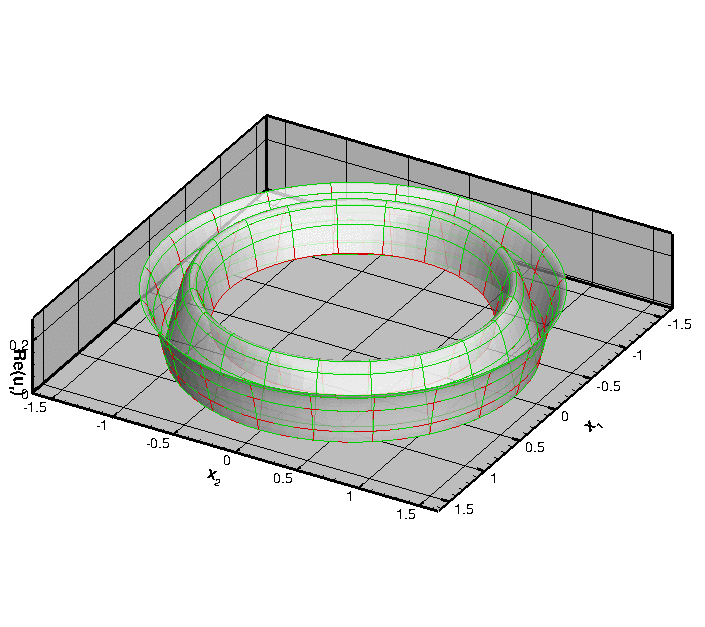Real part of the radial displacement. Green: exact; red: computed.

Finally, here is a plot of the real part of the horizontal displacement for the case when there is a 10% "gap" in the annular region. The presence of the gap clearly breaks the axisymmetry of the solution and creates waves that propagate in all directions: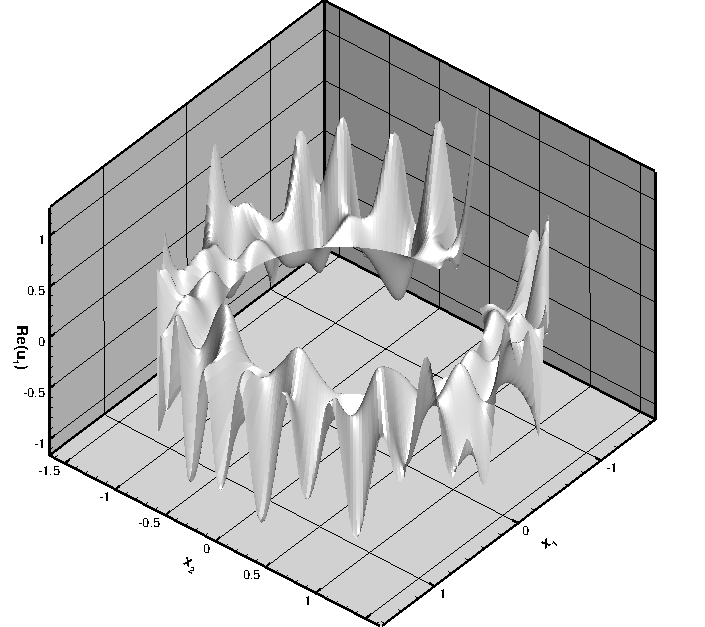Real part of the horizontal displacement for an incomplete annular region.

Unsurprisingly, we are not aware of an analytical solution for this problem.

# Global parameters and functions

As usual, we define all non-dimensional parameters in a namespace where we also define the displacement to be applied on the inner boundary, the traction (pressure) to be applied on the outer boundary, and the exact solution (which we don't list here because it is very lengthy).

//=======start_namespace==========================================
/// Global variables
//================================================================
{
/// Poisson's ratio
double Nu = 0.3;
/// Square of non-dim frequency
double Omega_sq=100.0;
/// The elasticity tensor
TimeHarmonicIsotropicElasticityTensor E(Nu);
/// Thickness of annulus
double H_annulus=0.5;
/// Displacement amplitude on inner radius
/// Real-valued, radial displacement field on inner boundary
void solid_boundary_displacement(const Vector<double>& x,
Vector<double>& u)
{
Vector<double> normal(2);
double norm=sqrt(x*x+x*x);
normal=x/norm;
normal=x/norm;
u=Displacement_amplitude*normal;
u=Displacement_amplitude*normal;
}
/// Uniform pressure
double P = 0.0;
/// Constant pressure load (real and imag part)
void constant_pressure(const Vector<double> &x,
const Vector<double> &n,
Vector<std::complex<double> >&traction)
{
unsigned dim = traction.size();
for(unsigned i=0;i<dim;i++)
{
traction[i] = complex<double>(-P*n[i],P*n[i]);
}
/////////////////////////////////////////////////////////////////// /////////////////////////////////...
double Displacement_amplitude
double H_annulus
Thickness of annulus.
double P
Uniform pressure.
void constant_pressure(const Vector< double > &x, const Vector< double > &n, Vector< std::complex< double > > &traction)
Constant pressure load (real and imag part)
void solid_boundary_displacement(const Vector< double > &x, Vector< double > &u)
Real-valued, radial displacement field on inner boundary.
TimeHarmonicIsotropicElasticityTensor E(Nu)
The elasticity tensor.
double Omega_sq
Square of non-dim frequency.

We also define the output directory and the number of elements in the mesh.

/// Output directory
string Directory="RESLT";
/// Number of elements in azimuthal direction
unsigned Ntheta=20;
/// Number of elements in radial direction
unsigned Nr=10;
unsigned Ntheta
Number of elements in azimuthal direction.
string Directory
Output directory.
unsigned Nr
Number of elements in radial direction.

# The driver code

We start by defining command line arguments which specify the number of elements in the mesh and indicate the presence or absence of the "gap" in the coating.

//=======start_of_main==================================================
/// Driver for annular disk loaded by pressure
//======================================================================
int main(int argc, char **argv)
{
// Store command line arguments
CommandLineArgs::setup(argc,argv);
// Define possible command line arguments and parse the ones that
// were actually specified
// Number of elements in azimuthal direction
CommandLineArgs::specify_command_line_flag(
"--ntheta",
// Number of elements in radial direction
CommandLineArgs::specify_command_line_flag(
"--nr",
// Do have a gap in the annulus?
CommandLineArgs::specify_command_line_flag("--have_gap");
int main(int argc, char **argv)
Driver for annular disk loaded by pressure.

The code performs a parameter study in which we compute the solution for a range of pressures. We specify the pressure increment and the number of steps to be performed, parse the command line arguments and document them.

// P increment
double p_increment=0.1;
CommandLineArgs::specify_command_line_flag("--p_increment",&p_increment);
// Number of steps
unsigned nstep=3;
CommandLineArgs::specify_command_line_flag("--nstep",&nstep);
// Parse command line
CommandLineArgs::parse_and_assign();
// Doc what has actually been specified on the command line
CommandLineArgs::doc_specified_flags();

Next, we create the problem (discretising the domain with nine-noded quadrilateral elements),

//Set up the problem

and perform the parameter study:

// Initial values for parameter values
//Parameter incrementation
for(unsigned i=0;i<nstep;i++)
{
// Solve the problem using Newton's method
problem.newton_solve();
// Doc solution
problem.doc_solution();
// Increment pressure
Global_Parameters::P+=p_increment;
}
} //end of main

# The problem class

The Problem class is very simple. As in other problems with Neumann boundary conditions, we provide separate meshes for the "bulk" elements and the face elements that apply the traction boundary conditions. The latter are attached to the relevant faces of the bulk elements by the function create_traction_elements().

//=============begin_problem============================================
/// Annular disk
//======================================================================
template<class ELASTICITY_ELEMENT>
class AnnularDiskProblem : public Problem
{
public:
/// Constructor:
/// Update function (empty)
/// Update function (empty)
void actions_before_newton_solve()
Update function (empty)
void actions_after_newton_solve()
Update function (empty)
/// Doc the solution
void doc_solution();
private:
/// Create traction elements
void create_traction_elements();
/// Delete traction elements
void delete_traction_elements();
/// Pointer to solid mesh
Mesh* Solid_mesh_pt;
/// Pointer to mesh of traction elements
Mesh* Traction_mesh_pt;
/// DocInfo object for output
DocInfo Doc_info;
};

# The problem constructor

We begin by building the "bulk" solid mesh, specifying the presence of the gap (and its width) if necessary. If there is no gap, the mesh is periodic; see Comments for a more detailed discussion of how the mesh for this problem is constructed.

//===========start_of_constructor=======================================
/// Constructor:
//======================================================================
template<class ELASTICITY_ELEMENT>
{
// Solid mesh
//-----------
// The mesh is periodic
bool periodic=true;
// Azimuthal fraction of elastic coating
double azimuthal_fraction_of_coating=1.0;
// Innermost radius for solid mesh
double a=1.0;
// Gap in annulus?
if (CommandLineArgs::command_line_flag_has_been_set("--have_gap"))
{
periodic=false;
azimuthal_fraction_of_coating=0.9;
}
// Build solid mesh
Solid_mesh_pt = new
RefineableTwoDAnnularMesh<ELASTICITY_ELEMENT>(
periodic,azimuthal_fraction_of_coating,

It is always a good idea to have a look at the mesh and its boundary enumeration:

// Let's have a look where the boundaries are
Solid_mesh_pt->output("solid_mesh.dat");
Solid_mesh_pt->output_boundaries("solid_mesh_boundary.dat");

We loop over the elements and specify the relevant physical parameters via the pointer to the tensor of constitutive parameters and the (square of the) non-dimensional frequency,

//Assign the physical properties to the elements
//Loop over the elements in the main mesh
unsigned n_element =Solid_mesh_pt->nelement();
for(unsigned i=0;i<n_element;i++)
{
//Cast to a solid element
ELASTICITY_ELEMENT *el_pt =
dynamic_cast<ELASTICITY_ELEMENT*>(Solid_mesh_pt->element_pt(i));
// Set the constitutive law
el_pt->elasticity_tensor_pt() = &Global_Parameters::E;
// Square of non-dim frequency
el_pt->omega_sq_pt()= &Global_Parameters::Omega_sq;
}

Next we create the mesh that contains the FaceElements that apply the traction boundary conditions, and combine all meshes into a global mesh:

// Construct the traction element mesh
Traction_mesh_pt=new Mesh;
create_traction_elements();
// Solid mesh is first sub-mesh
// Build combined "global" mesh
build_global_mesh();

We apply the displacement boundary conditions by pinning the real and imaginary part of the two displacement components at all nodes on boundary 0. We then impose a purely radial displacement on the real and imaginary parts of the displacement field, using the function defined in the Global_Parameters namespace:

// Solid boundary conditions:
//---------------------------
// Pin real and imag part of both displacement components
// on the inner (boundary 0)
unsigned b_inner=0;
unsigned n_node = Solid_mesh_pt->nboundary_node(b_inner);
//Loop over the nodes to pin and assign boundary displacements on
//solid boundary
Vector<double> u(2);
Vector<double> x(2);
for(unsigned i=0;i<n_node;i++)
{
Node* nod_pt=Solid_mesh_pt->boundary_node_pt(b_inner,i);
nod_pt->pin(0);
nod_pt->pin(1);
nod_pt->pin(2);
nod_pt->pin(3);
// Assign displacements
x=nod_pt->x(0);
x=nod_pt->x(1);
// Real part of x-displacement
nod_pt->set_value(0,u);
// Real part of y-displacement
nod_pt->set_value(1,u);
// Imag part of x-displacement
nod_pt->set_value(2,-u);
// Imag part of y-displacement
nod_pt->set_value(3,-u);
}

Finally we assign the equation numbers and specify the output directory:

//Assign equation numbers
cout << assign_eqn_numbers() << std::endl;
// Set output directory
Doc_info.set_directory(Global_Parameters::Directory);
} //end_of_constructor

# The traction elements

We create the face elements that apply the traction on the outer boundary (boundary 2). If there is a gap in the annular region we also apply the pressure loading on boundaries 1 and 3.

//============start_of_create_traction_elements==============================
/// Create traction elements
//=======================================================================
template<class ELASTICITY_ELEMENT>
{
// Load outer surface (2) and both "ends" (1 and 3) if there's a gap
unsigned b_lo=1;
unsigned b_hi=3;
// ...otherwise load only the outside (2)
if (!CommandLineArgs::command_line_flag_has_been_set("--have_gap"))
{
b_lo=2;
b_hi=2;
}
for (unsigned b=b_lo;b<=b_hi;b++)
{
// How many bulk elements are adjacent to boundary b?
unsigned n_element = Solid_mesh_pt->nboundary_element(b);
// Loop over the bulk elements adjacent to boundary b
for(unsigned e=0;e<n_element;e++)
{
// Get pointer to the bulk element that is adjacent to boundary b
ELASTICITY_ELEMENT* bulk_elem_pt = dynamic_cast<ELASTICITY_ELEMENT*>(
Solid_mesh_pt->boundary_element_pt(b,e));
//Find the index of the face of element e along boundary b
int face_index = Solid_mesh_pt->face_index_at_boundary(b,e);
// Create element
TimeHarmonicLinearElasticityTractionElement<ELASTICITY_ELEMENT>* el_pt=
new TimeHarmonicLinearElasticityTractionElement<ELASTICITY_ELEMENT>
(bulk_elem_pt,face_index);
// Associate element with bulk boundary (to allow it to access
// the boundary coordinates in the bulk mesh)
el_pt->set_boundary_number_in_bulk_mesh(b);
//Set the traction function
el_pt->traction_fct_pt() = Global_Parameters::constant_pressure;
}
}
} // end_of_create_traction_elements
void create_traction_elements()
Create traction elements.

# Post-processing

As expected, this member function documents the computed solution.

//==============start_doc===========================================
/// Doc the solution
//==================================================================
template<class ELASTICITY_ELEMENT>
{
ofstream some_file;
char filename;
// Number of plot points
unsigned n_plot=5;
// Output displacement field
//--------------------------
sprintf(filename,"%s/elast_soln%i.dat",Doc_info.directory().c_str(),
Doc_info.number());
some_file.open(filename);
Solid_mesh_pt->output(some_file,n_plot);
some_file.close();
// Output traction elements
//-------------------------
sprintf(filename,"%s/traction_soln%i.dat",Doc_info.directory().c_str(),
Doc_info.number());
some_file.open(filename);
Traction_mesh_pt->output(some_file,n_plot);
some_file.close();
// Output exact solution
//----------------------
sprintf(filename,"%s/exact_soln%i.dat",Doc_info.directory().c_str(),
Doc_info.number());
some_file.open(filename);
Solid_mesh_pt->output_fct(some_file,n_plot,Global_Parameters::exact_u);
some_file.close();
// Increment label for output files
Doc_info.number()++;
} //end doc
void doc_solution()
Doc the solution.
void exact_u(const Vector< double > &x, Vector< double > &u)
Exact solution as a Vector.

• We did not discuss the mesh generation in detail here: The mesh is created by straightforward overloading of oomph-lib's existing rectangular quad mesh – the constructor simply adjusts the nodal positions (exactly as suggested in the "Not-so-quick" guide.) A little bit of extra work is required to enforce periodicity on the mesh for the case without a gap in the annular region because two of the boundaries in the original mesh then overlap. How this is dealt with is discussed in another tutorial.

• If you inspect the driver code you will notice that it also contains relevant code to perform spatially adaptive simulations of the problem – the adaptive version of the code is selected with #ifdefs. Dealing with the periodic boundary conditions for spatially adaptive meshes requires a few additional steps, but they are also discussed elsewhere, so we won't discuss them here.

## Exercises

• Change the parameter study performed by the driver code such that the loop varies the frequency parameter. Assess the effect of an increase inon the accuracy of the solution by comparing the computed results against the exact solution at fixed spatial resolution.

• Explore the use of spatial adaptivity in the problem

• for the same parameter study suggested in the previous exercise (increase in)
and
• for the problem with the "gap" in the annular region.

• Modify the code to exploit at least some of the problem's symmetry, e.g. by solving the problem for the continuous annulus in a quarter of the original domain,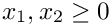, say, using appropriate symmetry boundary conditions along the coordinate axes.